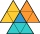# Length + The Law of Cosines - math problems

#### Number of problems found: 16

• Triangle's centroidIn the triangle ABC the given lengths of its medians tc = 9, ta = 6. Let T be the intersection of the medians (triangle's centroid) and point is S the center of the side BC. The magnitude of the CTS angle is 60°. Calculate the length of the BC side to 2 d
• Aircraft bearingTwo aircraft will depart from the airport simultaneously, the first with a course of 30° and the second with a course of 86°. Both fly at 330 km/h. How far apart will they be in 45 minutes of flight?What is the volume of a quadrilateral oblique prism with base edges of length a = 1m, b = 1.1m, c = 1.2m, d = 0.7m, if a side edge of length h = 3.9m has a deviation from the base of 20° 35' and the edges a, b form an angle of 50.5°.
• Two chordsFrom the point on the circle with a diameter of 8 cm, two identical chords are led, which form an angle of 60°. Calculate the length of these chords.
• Two grovesTwo groves A, B are separated by a forest, both are visible from the hunting grove C, which is connected to both by direct roads. What will be the length of the projected road from A to B, if AC = 5004 m, BC = 2600 m and angle ABC = 53° 45 ’?
• A rhombusA rhombus has sides of length 10 cm, and the angle between two adjacent sides is 76 degrees. Find the length of the longer diagonal of the rhombus.
• Children playgroundThe playground has a trapezoid shape, and the parallel sides have a length of 36 m and 21 m. The remaining two sides are 14 m long and 16 m long. Find the size of the inner trapezoid angles.
• ABCDAC= 40cm , angle DAB=38 , angle DCB=58 , angle DBC=90 , DB is perpendicular on AC , find BD and AD
• Inner anglesThe inner angles of the triangle are 30°, 45° and 105° and its longest side is 10 cm. Calculate the length of the shortest side, write the result in cm up to two decimal places.
• Medians of isosceles triangleThe isosceles triangle has a base ABC |AB| = 16 cm and 10 cm long arm. What is the length of medians?
• Diagonals in diamondIn the rhombus is given a = 160 cm, alpha = 60 degrees. Calculate the length of the diagonals.
• Scalene triangleSolve the triangle: A = 50°, b = 13, c = 6
• DiagonalsCalculate the length of the rhombus's diagonals if its side is long 5 and one of its internal angles is 80°.
• Two boatsTwo boats are located from a height of 150m above the surface of the lake at depth angles of 57° and 39°. Find the distance of both boats if the sighting device and both ships are in a plane perpendicular to the surface of the lake.
• Side cIn △ABC a=2, b=4 and ∠C=100°. Calculate length of the side c.
• Triangle and its heightsCalculate the length of the sides of the triangle ABC, if va=5 cm, vb=7 cm and side b is 5 cm shorter than side a.

Do you have an exciting math question or word problem that you can't solve? Ask a question or post a math problem, and we can try to solve it.

We will send a solution to your e-mail address. Solved examples are also published here. Please enter the e-mail correctly and check whether you don't have a full mailbox.

Do you want to convert length units? Cosine rule uses trigonometric SAS triangle calculator. Length - math problems. The Law of Cosines - math problems.